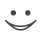# This flashcard is just one of a free flashcard set. See all flashcards!

46
The six-month zero rate is 8% per annum with semiannual compounding. The price of a one-year bond that provides a coupon of 6% per annum semiannually is 97. What is the one-year continuously compounded zero rate?
A.8.02%
B.8.52%
C.9.02%
D.9.52%

If the rate is R we must have

97= 3/1.04+103e^-R*1

or

e^-R= (97-3/1.04)/103 =0.9137

so that R = ln(1/0.9137) = 0.0902 or 9.02%.Flashcard info:
Author: CoboCards-User
Main topic: Finance & Investment
Topic: Derivatives
Published: 27.10.2015